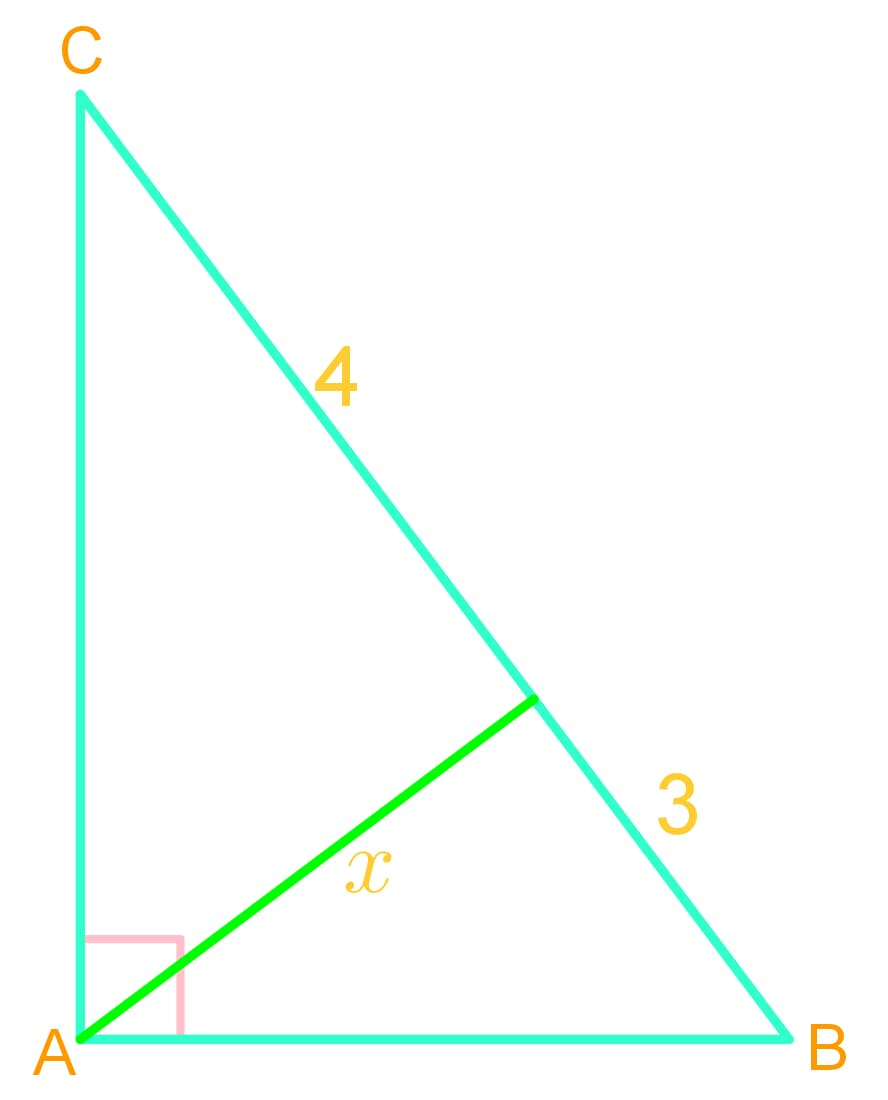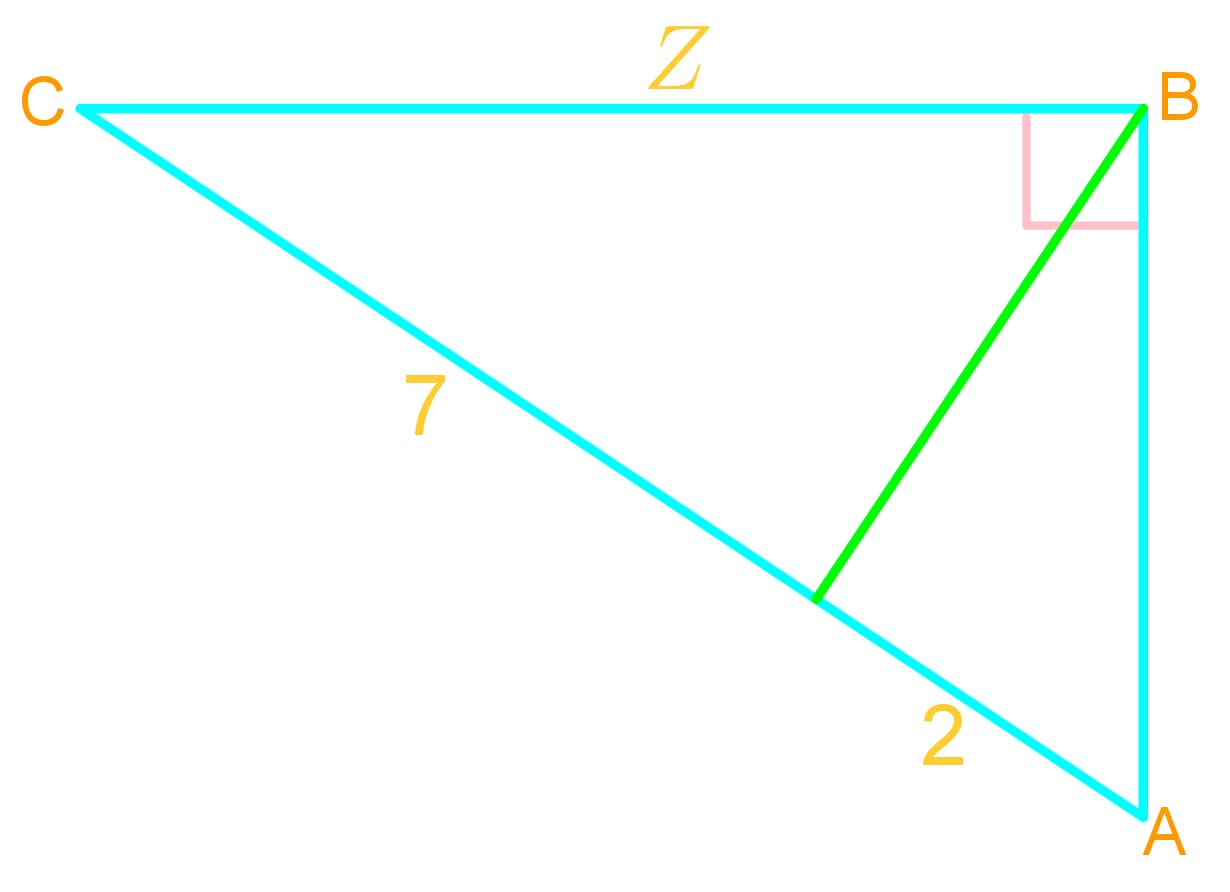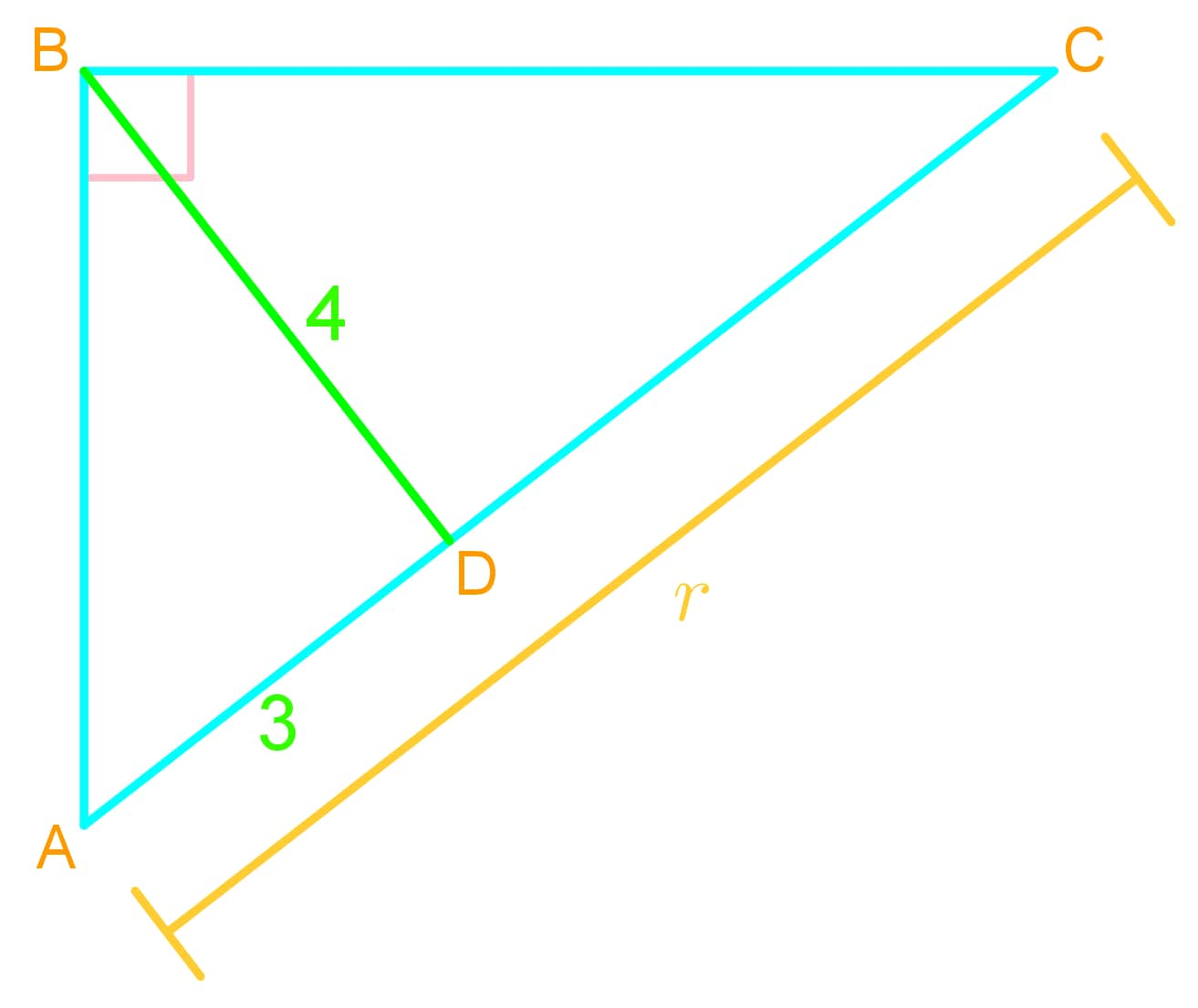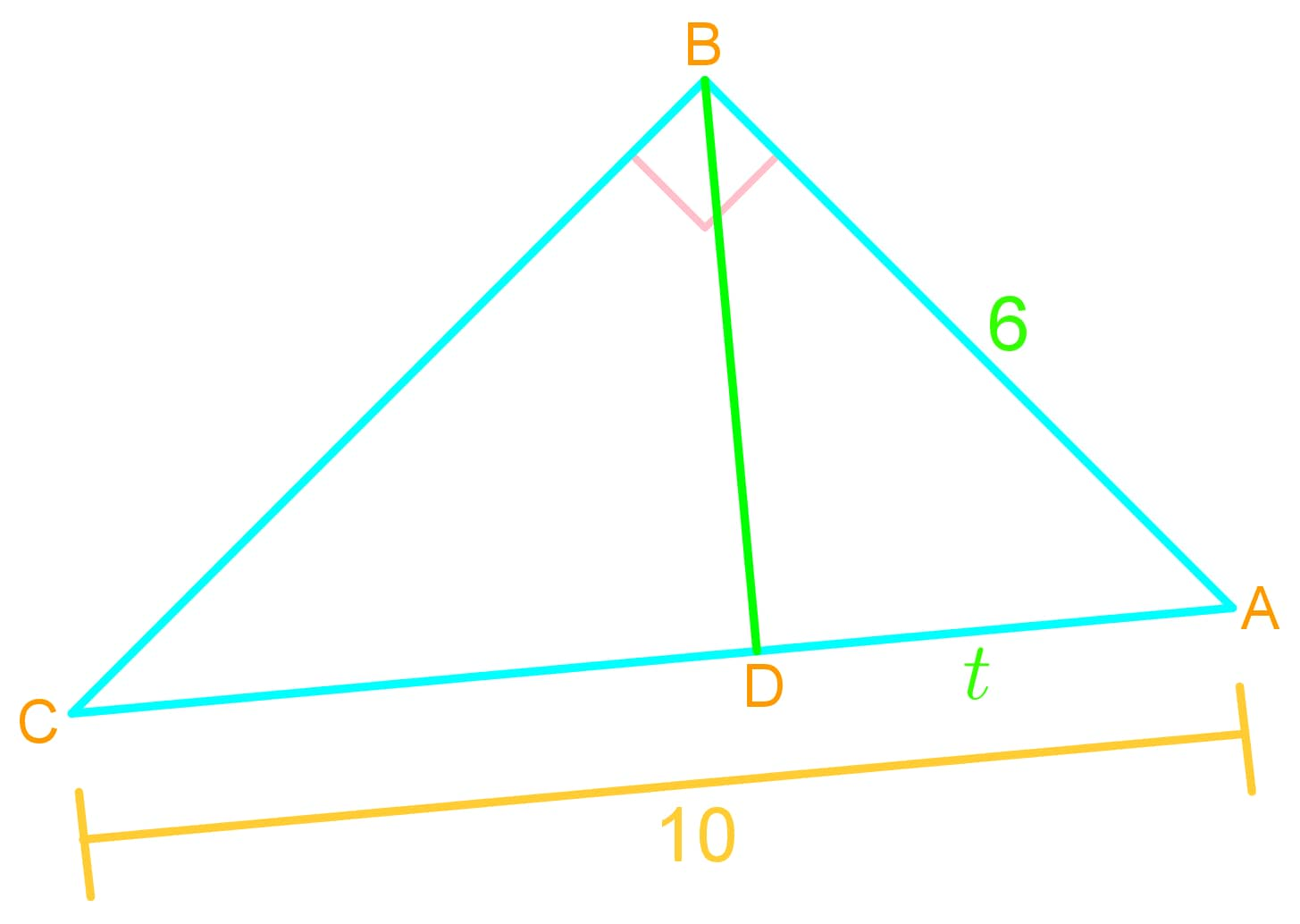# Arithmetic mean vs. Geometric mean

### Arithmetic mean vs. Geometric mean

#### Lessons

• Introduction
What are Arithmetic Mean and Geometric Mean?

• 1.
Determining the Arithmetic Mean of a Sequence
a)
What is the arithmetic mean between 2 and 8?

b)
What are the 3 arithmetic means between 8 and 18?

c)
What are the 4 arithmetic means between 22 and 6.5?

• 2.
Determining the Geometric Mean of a Sequence
a)
What is the geometric mean between 2 and 8?

b)
What is the geometric mean between 16 and 4?

• 3.
Applications of Geometric Mean in Geometry
Find the value of each variable in the following diagrams.
a)b)c)d)